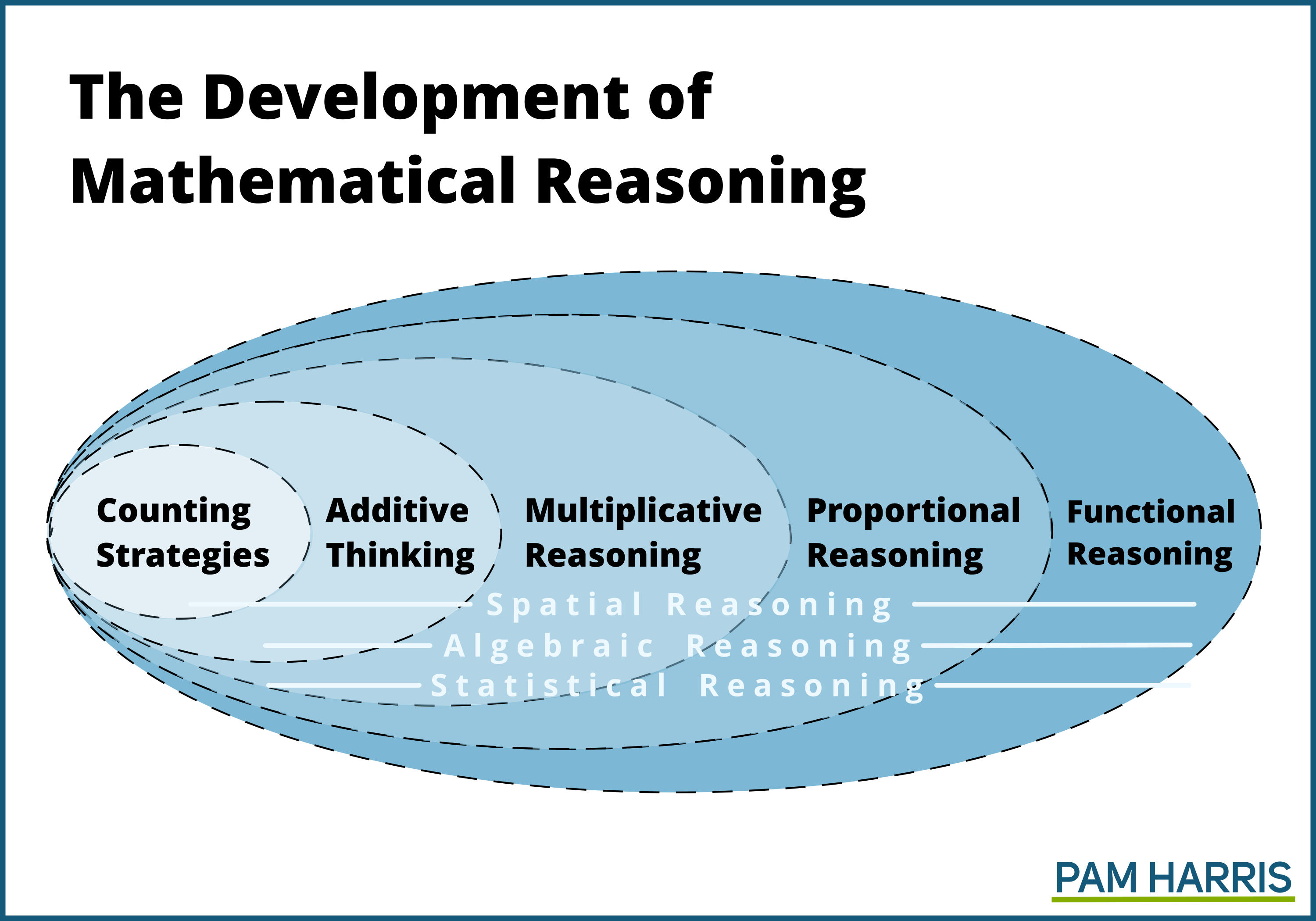# The Development of Mathematical Reasoning Part 3

Jul 17, 2020

## Answer Getting vs. Brain Building

Since I posted the graphic and blogs about the Development of Mathematical Reasoning, I have gotten questions.One of those questions is: But Pam, why isn’t it okay to teach students to do this thing (insert: algorithm, procedure, trick)? It works. Students get the right answer, it’s quick, it’s easy, it’s reliable. What’s wrong with using the reasoning in the level(s) before if students are getting the right answer? Isn’t that what we want, right answers?

Great question!

And I’ll ask a question back at ya:

If your goal is for students to do things to get correct answers, then everything is on the table. Whatever works is fair game: rhymes, raps, stories, a clever mnemonic, steps that don’t require much reasoning at all. If it gets a right answer and students can do the steps, then let’s use it, right?

But if your goal is to help students build their brains so that they create more mental relationships, literally changing students’ brain structure so that they have more mental connections, then you want to encourage students to always be building toward more sophisticated thought. If your goal is mathematizing, you want to help students wrestle with the problem, making sense out of the problem and the relationships. You want to purposefully cause disequilibrium so that students seek to resolve that disequilibrium by figuring out what is happening. You want to ask questions that students have access to but that nudge students to use increasingly more complicated relationships – as they learn to deal with more and more, they learn—not just to get answers but to think differently, more complexly. This is my goal.

### Here are a few examples:

If we teach the traditional algorithm for addition and subtraction, we run the risk that students solve the problems using counting strategies. Even if students are using additive thinking for each of the column addition, the biggest numbers they’ll ever deal with additively are single digits. Even if students are using additive reasoning when subtracting in each column, the biggest numbers they’ll ever deal with are teens subtracting single digits. We need students to wrestle additively with bigger numbers.

If we teach the traditional algorithm for multiplication, we run the risk that students solve the problems using additive reasoning, skip counting to find each single digit multiplication. At best, multiplying with the traditional algorithm affords a student to reason multiplicatively but only with single digit facts. We need students to wrestle multiplicatively with bigger numbers.

If we teach students the traditional algorithm for division, we almost certainly work against rational number sense. How? Consider a problem like 1188 divided by 12. The first step in the traditional algorithm has you asking, how many times does 12 go into (divide into) 1. We usually say, “Nope,” and move onto asking how many times 12 goes into 11, and so forth. Consider that question: how many times does 12 divide into 1? Another way of asking that question is to consider that 12 times something is 1, 12 × ___ = 1. And the answer in the algorithm is “Nope.”  But isn’t there something that goes in that blank? Isn’t there a number that when multiplied by 12 gives 1? What about 1/12? Isn’t 12 × 1/12 = 1? I submit that teaching the traditional algorithm often actually harms students’ rational number sense. It’s almost like when students are learning the division algorithm, we tell them there is no answer to 12 × ___ = 1, but then in middle school we say that there is an answer. That’s a rule that expires. We need to banish rules that expire.

If we teach students to cross multiply and divide to solve proportions, then students are using multiplication and division, multiplicative reasoning, to solve proportional reasoning problems. That’s reasoning from the level before. Instead we need students to grapple with the ratio and equivalent ratios, with how scaling one value in a ratio affects the ratio, what it means to scale in tandem.

All too often, the goal of mathematics teachers has been to help their students get answers to problems. What would happen, could happen if instead the goal of mathematics teachers is to help build students’ brains, help students create a neural network of relationships, so that students could think and reason more and more sophisticatedly?

I’m grateful to the mathematics educator and author of the Common Core State Standards for mathematics, Phil Daro, for helping me understand and find words to describe these different goals. In the video above I discussed Phil Daro’s finding from several years of TIMMS studies where he analyzed video from around the world of 8th grade mathematics classrooms.

Here is Phil Daro himself discussing answer getting versus developing reasoning.

Close